Next: 8.4 The Ruler Function Up: 8. Integrable Functions Previous: 8.2 Properties of the   Index

# 8.3 A Non-integrable Function

We will now give an example of a function that is not integrable. Let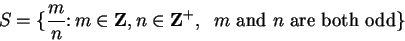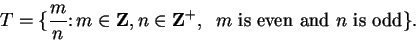Then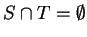, since if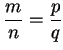where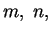and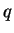are odd and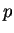is even, then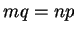which is impossible since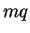is odd and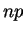is even.

8.35   Lemma. Every interval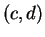in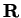with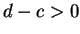contains a point in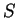and a point in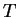.

Proof: Sincewe can choose an odd integer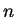such that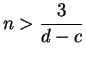, i.e.,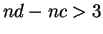. Since the interval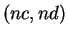has length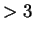, it contains at least two integers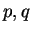, say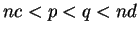. Ifandare both odd, then there is an even integer between them, and ifandare both even, there is an odd integer between them, so in all cases we can find a set of integers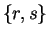one of which is even and the other is odd such that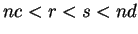, i.e.,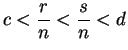. Then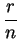and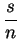are two elements ofone of which is in, and the other of which is in.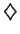8.36   Example (A non-integrable function.) Letbe defined by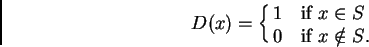(8.37)

I will find two partition-sample sequences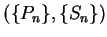and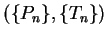such that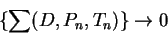and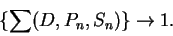It then follows that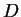is not integrable. Let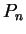be the regular partition of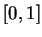intoequal subintervals.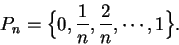Let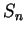be a sample forsuch that each point inis inand let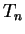be a sample forsuch that each point inis in. (We can find such samples by lemma 8.35.) Then for all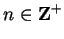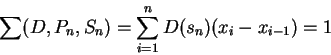and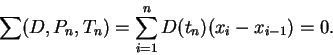So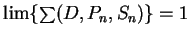and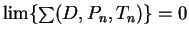. Bothandare partition-sample sequences for, so it follows thatis not integrable.

Our example of a non-integrable function is a slightly modified version of an example given by P. G. Lejeune Dirichlet (1805-1859) in 1837. Dirichlet's example was not presented as an example of a non-integrable function (since the definition of integrability in our sense had not yet been given), but rather as an example of how badly behaved a function can be. Before Dirichlet, functions that were this pathological had not been thought of as being functions. It was examples like this that motivated Riemann to define precisely what class of functions are well enough behaved so that we can prove things about them.Next: 8.4 The Ruler Function Up: 8. Integrable Functions Previous: 8.2 Properties of the   Index
Ray Mayer 2007-09-07